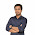Saturday, January 4, 2014

SPOJ DCEPC206

Problem link: SPOJ Problem Set (classical): 10810. Its a Murder!

Given a sequence of integers (at most 105 integers ranging from 0 to 106. You have to go from left to right, and for each position, you have to add the sum of all the numbers before current position which are strictly smaller than the number in current position.

Well, if the numbers where sorted, then we could do it more easily, i.e. keep an array for cumulative sum, and then calculate the answer in O(n) time. However, this is not the case. So, what can we do? According the problem, you have to maintain the cumulative sum anyway. So for each number in the sequence, if we keep adding them one by one, we can break down the problem in two parts. Let's think of a data structure which keeps cumulative sums, and we can add numbers anywhere and still maintain the sum. Segment tree / or binary indexed tree could come handy in this situation, where, the later one is more straight forward and less memory hungry. Now the steps are:

1 ⇒ Query: When a number is to be added, what is the sum of numbers smaller than current one? Note, as we are adding one by one in the order they appear, so the question whether there are any numbers which came after current number is eliminated. It is guaranteed that, when you are adding the ith number, all the numbers already present in the data-structure came before position i.

2 ⇒ Update: Once you have taken the partial sum for current number, it is now time to add the number in proper place, and update the cumulative sum structure. Here, clearly, appropriate position means, the position where the number would be placed when sorted. Now, we can do two things here. (a), we can sort the number and give each number an unique id to indicate its position in the sorted array. And (b), as the numbers are up to 106, we can use the numbers as their indices or positions in the sorted array. It is faster and the memory overhead is not that of a big issue here.

Now, here are two more things to be taken care about, (a), there can be duplicate numbers, and (b), 0 can be present in the input sequence, which can cause some trouble if you use BIT. However, (a) is handled naturally, because the problem wanted you to add only strictly smaller numbers, so it doesn't matter if you add same numbers in the same positions. There will be no query points in-between the equal numbers. And for (b), there is really no need to worry about 0, cause it contributes nothing at all, just ignore 0.

So, the overall algorithm looks like the following:

```for each number ai:
if ai > 0:
ans += BIT_Query(ai - 1)
BIT_Update(ai, ai)
```

Here: BIT_Query(pos) returns the sum up to position pos and BIT_Update(pos, val) adds val in pos in the BIT structure. Solutions involving the segment tree is also similar. Just keep the tree of total sums, whenever you are trying to add a number, read the partial sum, and then update the structure.

Happy coding..

1 comment:

1.Sir can you please explain the above concept with an example?